## Coming soon: Shape, tables and square numbers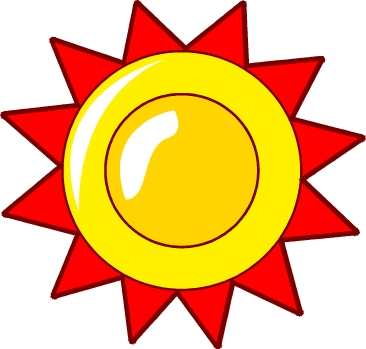How well are tables known? Here is a quick sheet to see how well the 3x, 4x, 6x, 8x and 10 times tables are known. Probably the two hardest of these are the 6 times and 8 times tables.

I have a nice little shape investigation, suitable for year 2. Circles, triangles, rectangles and hexagons can be bought in the shape shop. They are priced according to the number of sides. Circles cost 1p hexagons 6p etc.

What shapes can be bought for 20p?

A good challenge which uses knowledge of shape, counting money and logical thinking.

By Year 6 children are expected to know the square of numbers up to at least 10. next week we have two pages which  provide some revision of this together with some extension work on finding square numbers of multiples of 10. Suitable for the Year 6 Planning for Block B Unit 3 Week 2.

## How to find prime factors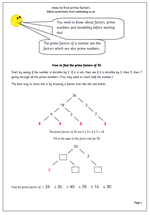Here we have a maths worksheet for year 6 on how to find prime factors. Before attempting this page it is necessary for children to have a good understanding of factors and prime numbers.

Finding prime factors is often done in the form of a diagram, rather like a tree diagram with the numbers at the bottom all being the prime factors. Frequently the same number is found more than once. For example the prime factors of 36 are 3, 3, 2 and 2.

A good way of checking if all the factors have been found is to multiply them and the original number should result. eg 3 x 3 x 2 x 2 = 36

This page is found in our Year 6 calculating section but has also been included in the Block planning resources:

Year 6 Block B Unit 3 Week 1: Securing number facts

How to find prime factors

## Resource of the Week: Time to the quarter hour.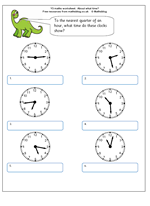This maths worksheet on reading time to the nearest quarter of an hour highlights several issues which create problems for children.

Firstly, on the clock face the hours are clearly numbered but the minutes are not and children need to be able to count on in fives before they can read minutes successfully.

Secondly, when telling someone the time we often approximate, either to the nearest quarter of an hour or the nearest five minutes, even when we can see clearly what the time is to the nearest minute.

Thirdly, when saying the time out or writing it we use several different conventions, as shown on the answer sheet.

Lastly, many children seldom come across this type of clock face, especially if they use digital watches, mobile phones etc., therefore take much longer to work it out – don’t be surprised to find ten year olds unable to read an analogue clock correctly.

## Year 1 maths worksheet: Change from 10p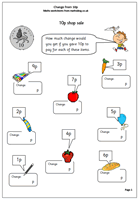Thanks to urbrainy.com for this money worksheet, suitable for year 1 children. It’s always a good idea to set up a little shop at home and take turns buying and selling items. There is an amazing amount of maths involved in this, from learning how to write numbers to counting on and back and finding two or three lots of a number. If a shop is not available why not try this page?

Change_from_10p

## Calculator game: Multiples of 4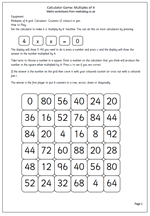Once children have learned the 4 times table this calculator game can be played. Set up the calculator to be a 4 times table machine by pressing 4 x x = 0 and then all you have to do to multiply a number by 4 is to type the number and press equals (don’t delete the answer before pressing the next number). Choose a number on the grid and say it out loud, then try and make that number by entering a number on the calculator and multiplying it by 4 by pressing =. If correct place a counter on the grid. Continue taking in turns with the aim of getting 4 in a row.

Complicated? Why not try the game and see just how easy it is.

Multiples of 4_larger numbers

## Coming soon: Money, prime factors and multiples of 4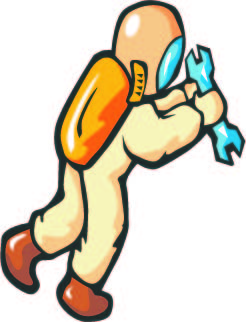Thanks to urbrainy.com for next week’s money worksheet, suitable for year 1 children. It’s always a good idea to set up a little shop at home and take turns buying and selling items. There is an amazing amount of maths involved in this, from learning how to write numbers to counting on and back and finding two or three lots of a number. If a shop is not available why not try this page?

For those who enjoyed the Year 3 calculation game on the 4x table there is a follow up with harder multiples of 4 which really test knowledge of the 4x table.

We will also have a maths worksheet for year 6 on how to find prime factors. Before attempting this page it is necessary for children to have a good understanding of factors and prime numbers.

## Year 6 maths worksheet: Decimal fractions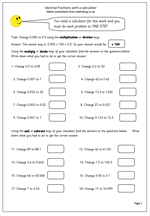A calculator is needed for this page as changes have to be made to decimals, using just one step. This is a good practice page for understanding multiplying and dividing decimals by 10, 100 or 1000, as well as some nifty mental arithmetic adding and subtracting.  It is the second of our pages for the Year 6 plans for Counting, partitioning and calculating. (Block A Unit 3 Week 2) which most schools wil be following next week.

This page can be found in our Year 6 Plannning resources as well as in the Understanding Number section.

Decimals with a calculator (1)

## Year 4 Calculations: Multiplication with words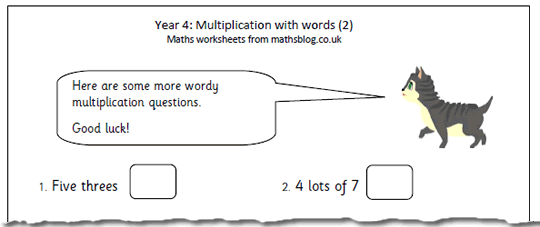Here we have the second page which looks at the vocabulary used for multiplication questions. This can used as a quick check to see how well the language is understood as well as how well tables are known. Terms such as ‘lots of’, ‘multiply by’, ‘times’, ‘multiplied by’ are all important to know.

This can be found in our year 4 Calculations section but there are also a lot of other multiplication pages in our Four Rules section.

Multiplication_with_words_(pg 2)

## Year 5 maths worksheet: Rounding to 1000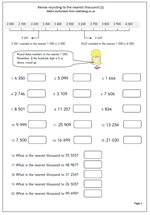Many people criticise the fact that children use calculators and, indeed, they have huge potential for giving incorrect answers: numbers left in the memory and mishit keys are just two ways of ensuring wrong answers. That is why it is so important that a mental calculation, no matter how brief, always goes on at the same time as the calculator is used, to ensure that the answer is ‘sensible’.

For example, a child might want to know quickly what 3 876 x 3 is. By all means use the calculator, but at the same time, he should be rounding 3 876 to 4 000 and multiplying 4 000 by 3 to make 12 000. This should take only a second or so to do in his head. He then knows that the answer will be just less than 12 000.

3 876 x 3 is actually 11 628

This is why the ability to round quickly and sensibly is so important. Hence this worksheet, found in our Year 5 Counting and Number section.

Round to the nearest_1000

## Calculator game: 4x table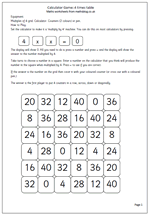Here is another in our series of calculator activities for year 3 children. It is a two player game intended to help with reinforcing the 4 times table. Calculators for young children have been frowned upon over the last few years but they can be a powerful tool for learning and should not be ignored as a resource. We will be following this up with a similar ‘multiples of 4’ page in a couple of weeks time.

4x table calculator game